# 0 -2 - The matrix A -11 2 2 -1 has eigenvalues 5 X = 3,...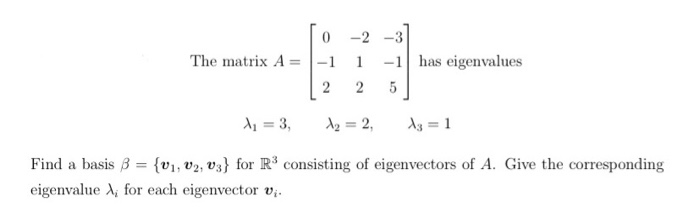0 -2 - The matrix A -11 2 2 -1 has eigenvalues 5 X = 3, A2 = 2, 13 = 1 Find a basis B = {V1, V2, v3} for R3 consisting of eigenvectors of A. Give the corresponding eigenvalue for each eigenvector vi.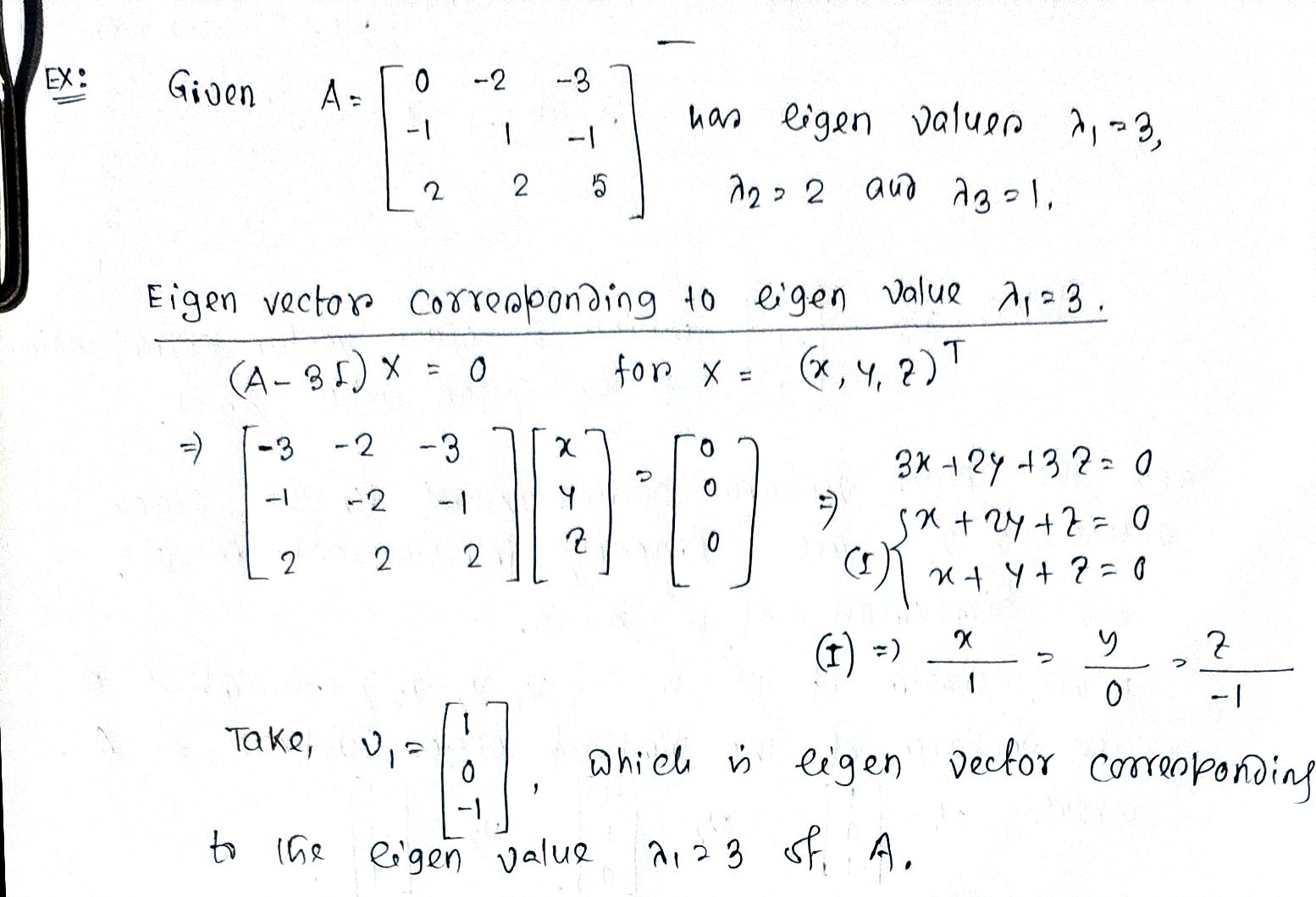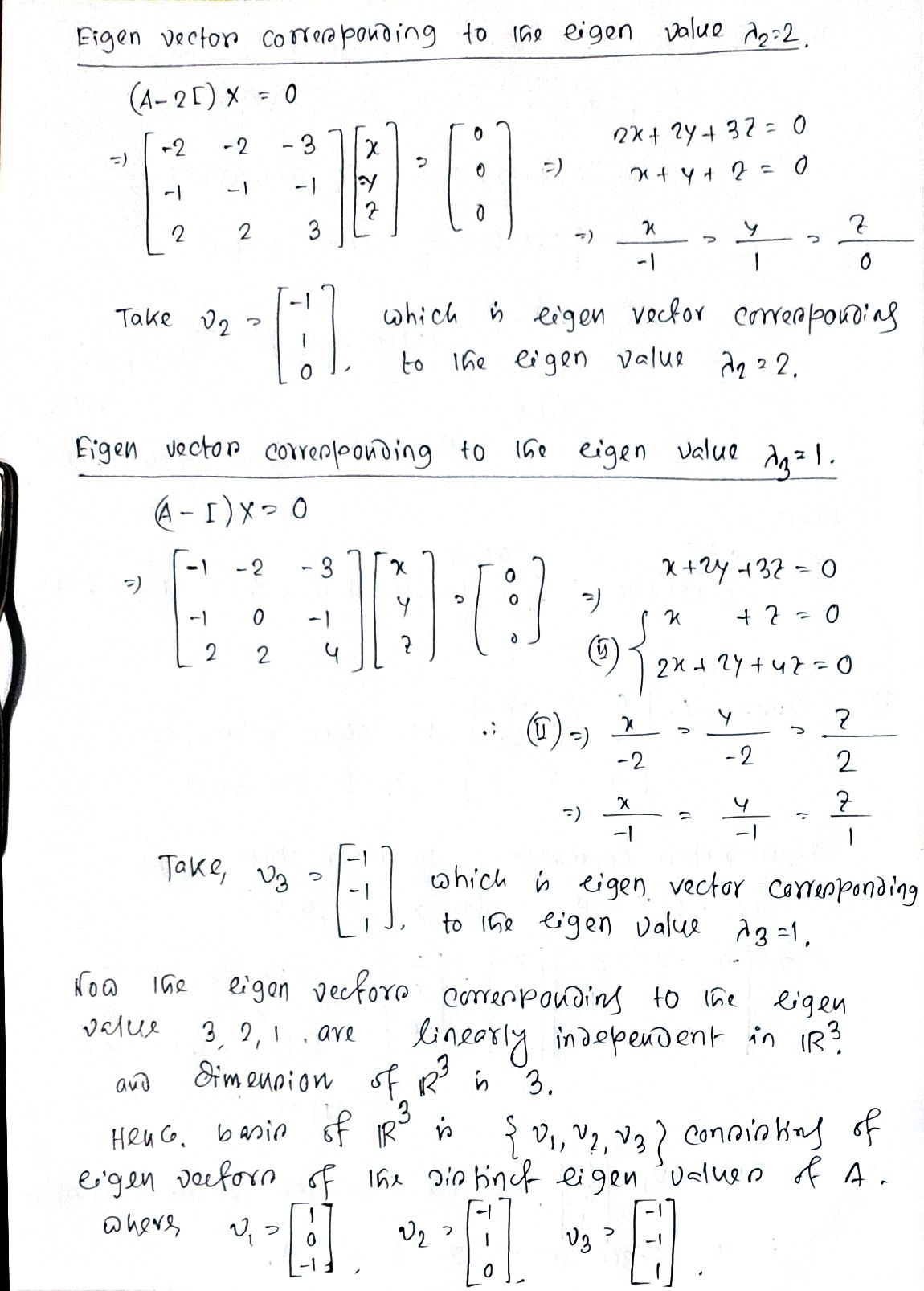##### Add Answer of: 0 -2 - The matrix A -11 2 2 -1 has eigenvalues 5 X = 3,...
Similar Homework Help Questions
• ### 8. Find a symmetric 3 x 3 matrix with eigenvalues 11, 12 , and , 13...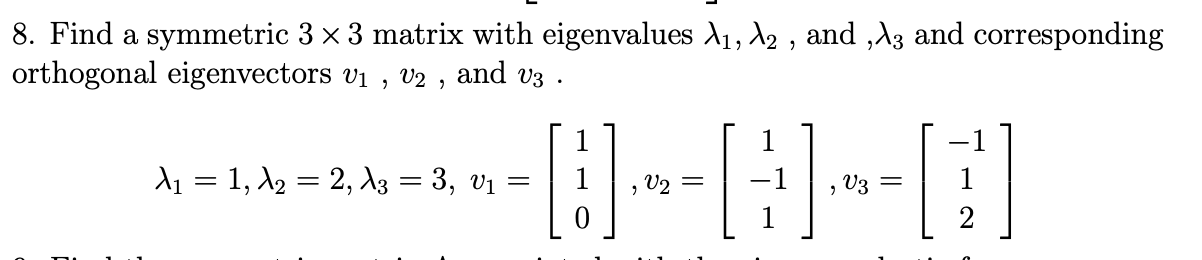8. Find a symmetric 3 x 3 matrix with eigenvalues 11, 12 , and , 13 and corresponding orthogonal eigenvectors vi , V2 , and V3 1 11 = 1, 12 = 2, 13 = 3, vi -=[:)--[:)--[;)] 1

• ### (Only need help with parts b and c) Consider the transition matrix If the initial state is x(0) =...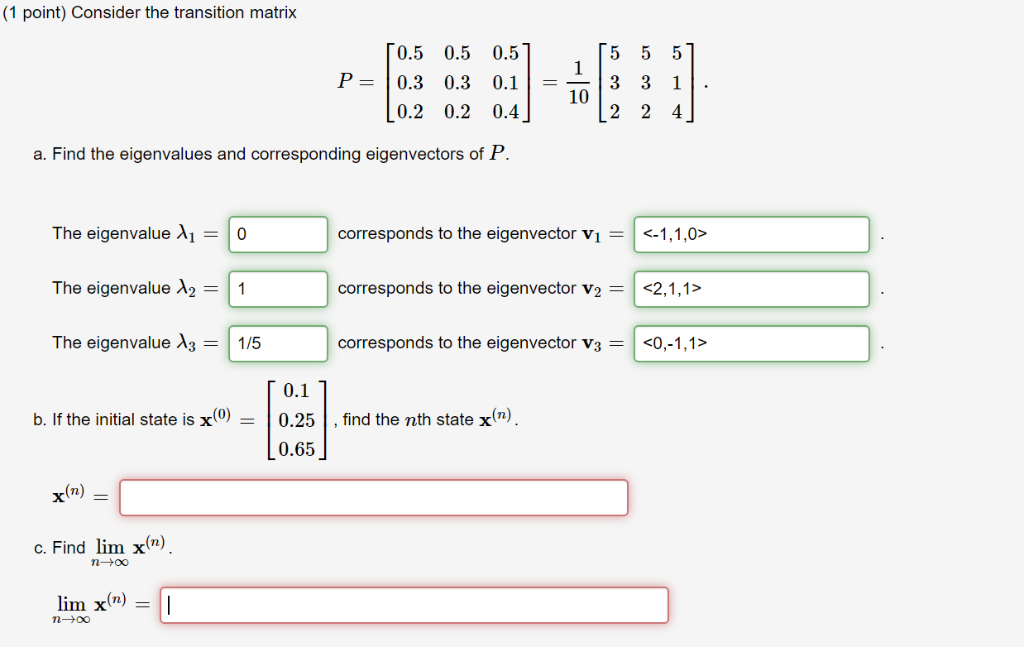(Only need help with parts b and c) Consider the transition matrix If the initial state is x(0) = [0.1,0.25,0.65] find the nth state of x(n). Find the  limn→∞x(n) (1 point) Consider the transition matrix 0.5 0.5 0.5 P 0.3 0.3 0.1 0.2 0.2 0.4 10 a. Find the eigenvalues and corresponding eigenvectors of P. ,-| 0 The eigenvalue λι The eigenvalue λ2-1 The eigenvalue A3 1/5 corresponds to the eigenvector vi <-1,1,0> corresponds to the eigenvector v2 = <2,1,1>...

• ### 4. (15 pts Consider the following direction fields IV VI (5 pts)Which of the direction fields corresponds to the system x -Ax, where A is a 2x2 matrix with eigenvalues λ,--1 and λ2-2 and correspondin...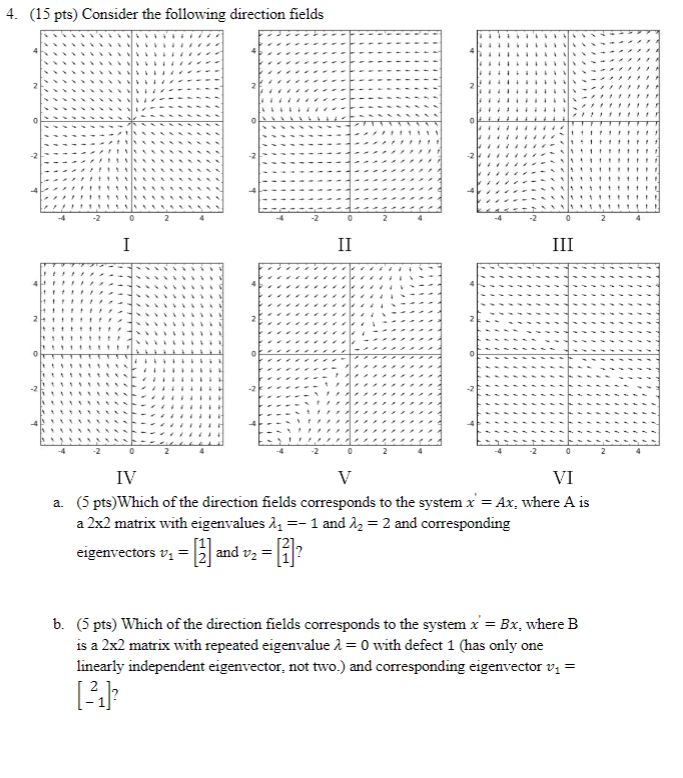4. (15 pts Consider the following direction fields IV VI (5 pts)Which of the direction fields corresponds to the system x -Ax, where A is a 2x2 matrix with eigenvalues λ,--1 and λ2-2 and corresponding eigenvectors vand v- 1? a. is a 2x2 matrix with repeated eigenvalue λ = 0 with defect 1 (has only one linearly independent eigenvector, not two.) and corresponding eigenvector vi- 13 (5 pts) Which of the direction fields corresponds to the system x -Cx, where...

• ### Let 4-β 0 0 A=1 0 4-3 024-β where β > 0 is a parameter. (a) Find the eigenvalues of A (note the eigenvalues will be functions of β). (b) Determine the values of β for which the matrix A is positiv...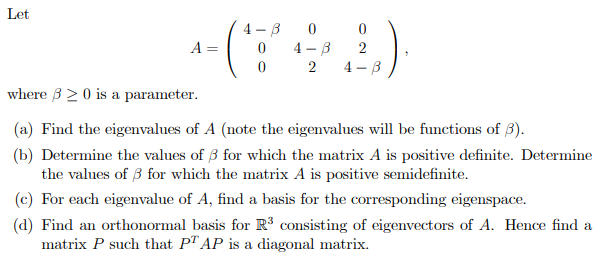Let 4-β 0 0 A=1 0 4-3 024-β where β > 0 is a parameter. (a) Find the eigenvalues of A (note the eigenvalues will be functions of β). (b) Determine the values of β for which the matrix A is positive definite. Determine the values of β for which the matrix A is positive semidefinite. (c) For each eigenvalue of A, find a basis for the corresponding eigenspace. (d) Find an orthonormal basis for R3 consisting of eigenvectors of...

• ### (1 point) Find the characteristic polynomial of the matrix 5 -5 A = 0 [ 5...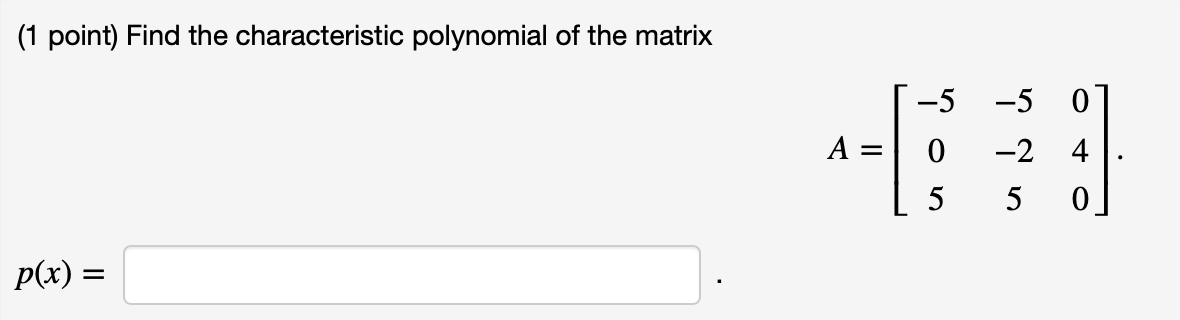(1 point) Find the characteristic polynomial of the matrix 5 -5 A = 0 [ 5 -5 -2 5 0] 4. 0] p(x) = (1 point) Find the eigenvalues of the matrix [ 23 C = -9 1-9 -18 14 9 72 7 -36 : -31] The eigenvalues are (Enter your answers as a comma separated list. The list you enter should have repeated items if there are eigenvalues with multiplicity greater than one.) (1 point) Given that vi =...

• ### 1. (20 points) For each of the statements, circle whether it is true or false. (a)...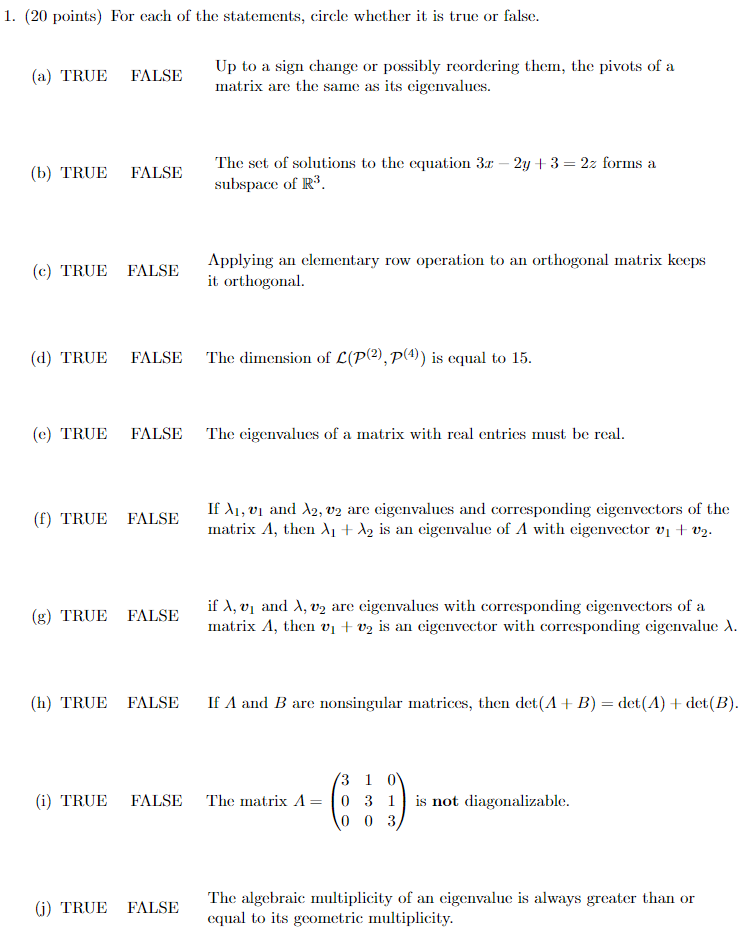1. (20 points) For each of the statements, circle whether it is true or false. (a) TRUE FALSE Up to a sign change or possibly reordering them, the pivots of a matrix are the same as its eigenvalues. (b) TRUE FALSE The set of solutions to the equation 3.0 - 2y +3 = 2z forms a subspace of R3 (c) TRUE FALSE Applying an elementary row operation to an orthogonal matrix keeps it orthogonal. (d) TRUE FALSE The dimension of...

• ### Matrix Question!eigenvectors and eigenvalues for symmetric matrix?

Suppose A is a symmetric N X N matrix with eigenvectors vi, i = 1; 2; 3 ...N withcorresponding eigenvalues ?i, i = 1; 2; 3 ...N.Pick any two distinct eigenvalues (assuming such a pair exists). Let's call them ?1 and?2 and their corresponding eigenvectors v1 and v2.Write down the matrix equations that show that v1 and v2 are eigenvectors of A.

• ### Suppose that the matrix A A has the following eigenvalues and eigenvectors: (1 point) Suppose that...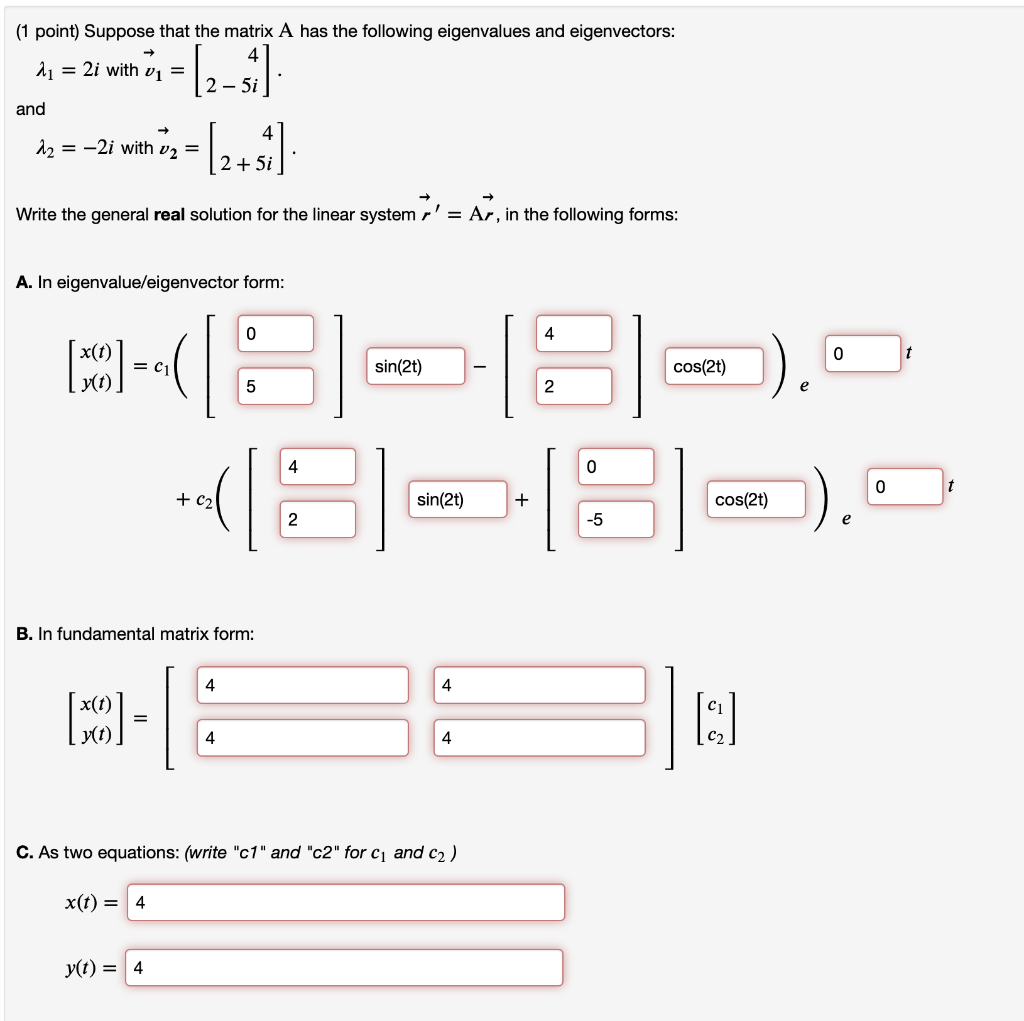Suppose that the matrix A A has the following eigenvalues and eigenvectors: (1 point) Suppose that the matrix A has the following eigenvalues and eigenvectors: 2 = 2i with v1 = 2 - 5i and - 12 = -2i with v2 = (2+1) 2 + 5i Write the general real solution for the linear system r' = Ar, in the following forms: A. In eigenvalue/eigenvector form: 0 4 0 t MODE = C1 sin(2t) cos(2) 5 2 4 0 0...

• ### (1 point) Suppose that the matrix A has the following eigenvalues and eigenvectors: 4 = 2...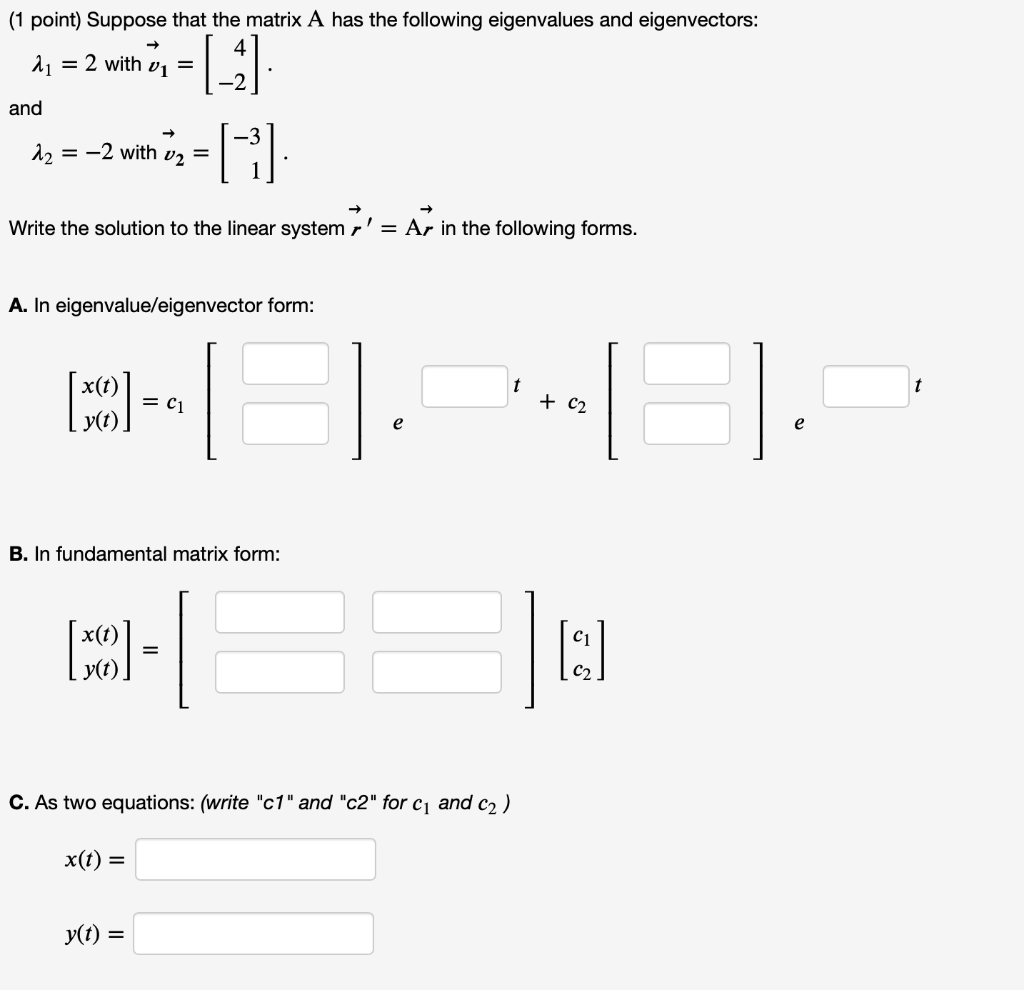(1 point) Suppose that the matrix A has the following eigenvalues and eigenvectors: 4 = 2 with vi = and |_ G 12 = -2 with v2 = Write the solution to the linear system r' = Ar in the following forms. A. In eigenvalue/eigenvector form: x(t) (50) = C1 + C2 e e B. In fundamental matrix form: (MCO) = I: C. As two equations: (write "c1" and "c2" for C1 and c2) x(t) = yt) =

• ### a) suppose that the nxn matrix A has its n eigenvalues arranged in decreasing order of absolute size, so that  >>....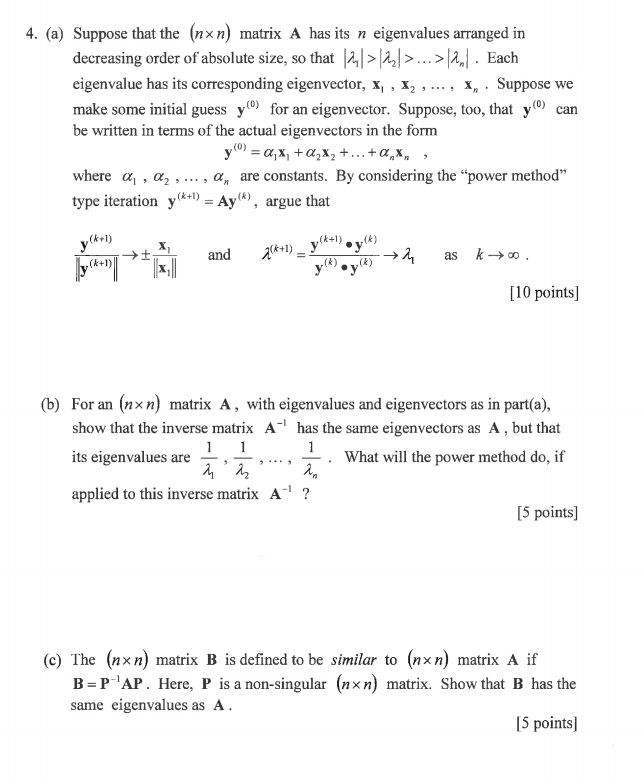a) suppose that the nxn matrix A has its n eigenvalues arranged in decreasing order of absolute size, so that  >>.... each eigenvalue has its corresponding eigenvector, x1,x2,...,xn. suppose we make some initial guess y(0) for an eigenvector. suppose, too, that y(0) can be written in terms of the actual eigenvectors in the form y(0)=alpha1.x1 +alpha2.x2 +...+alpha(n).x(n), where alpha1, alpha2, alpha(n) are constants. by considering the "power method" type iteration y(k+1)=Ay(k) argue that (see attached image) b) from an nxn...

Free Homework App